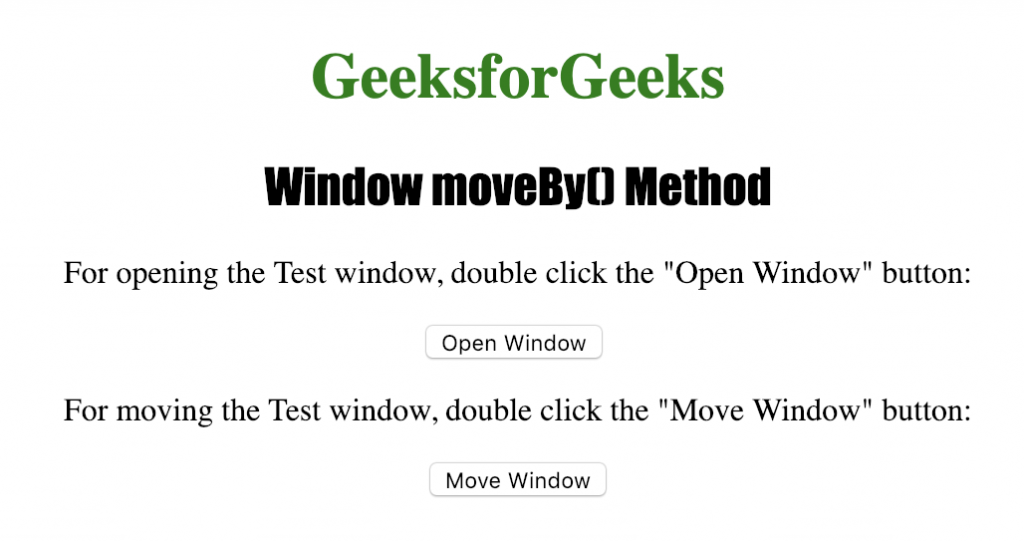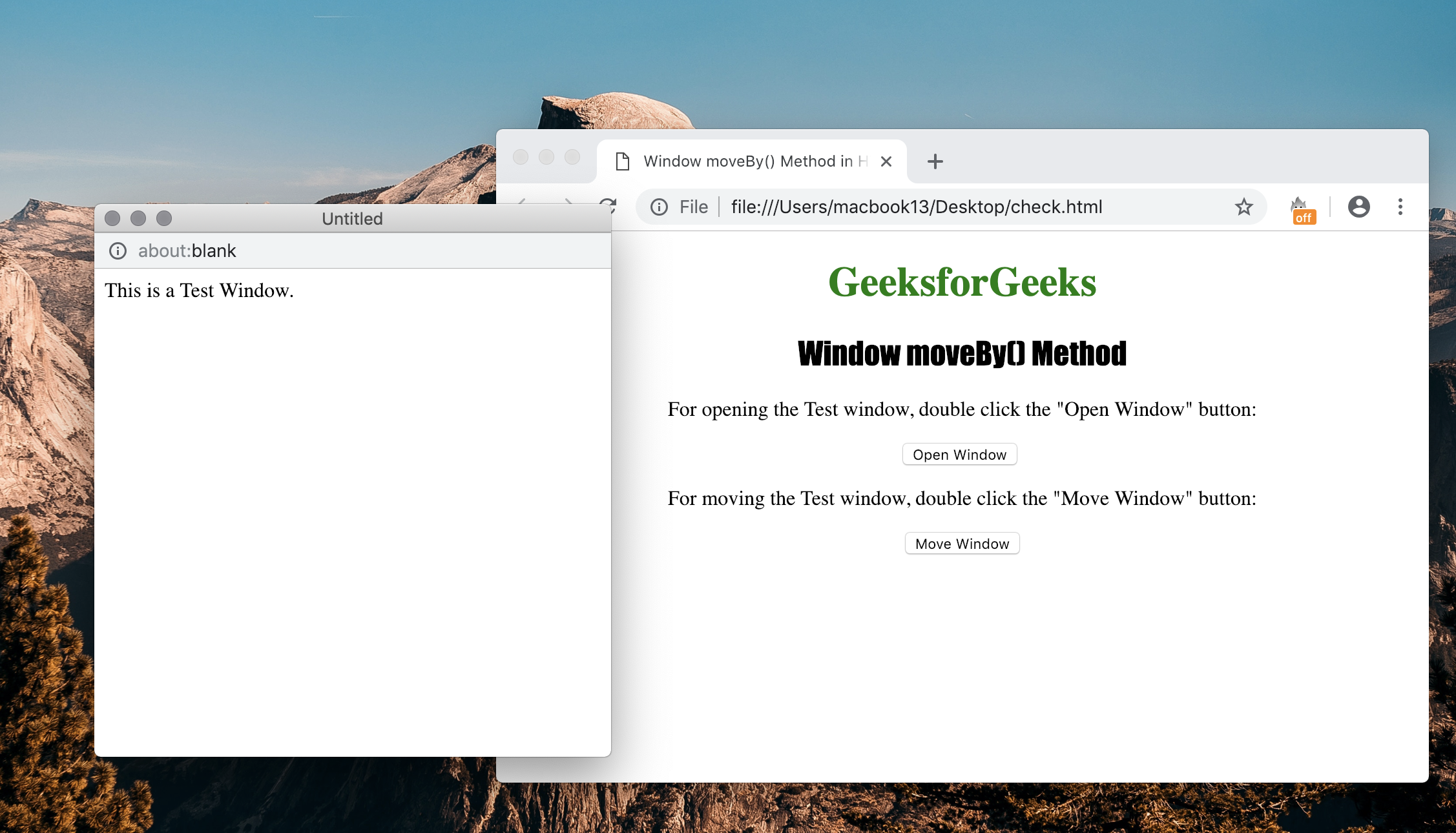# HTML | Window moveBy() Method

• Last Updated : 01 Jun, 2022

The Window moveBy() method is used for moving a window with a specified number of pixels relative to its current coordinates. The Window moveBy() method accepts two parameters x and y which denote the number of pixels to move the window horizontally and vertically.

Syntax:

`window.moveBy(x, y)`

Parameters Used:

• x : It is a mandatory parameter that specifies the amount of pixels to move the window horizontally.
• y : It is a mandatory parameter that specifies the amount of pixels to move the window vertically.

Below program illustrates the Window moveBy() Method :

Program: Moving a new window relative to its current position

## HTML

 ```<``html``>` `<``head``>``    ``<``title``>``      ``Window moveBy() Method in HTML``    ````    ``<``style``>``        ``h1 {``            ``color: green;``        ``}``        ` `        ``h2 {``            ``font-family: Impact;``        ``}``        ` `        ``body {``            ``text-align: center;``        ``}``    ````` `<``body``>` `    ``<``h1``>GeeksforGeeks``    ``<``h2``>Window moveBy() Method` `    ``<``p``>``      ``For opening the Test window,``      ``double click the "Open Window" button:``    ``` `    ``<``button` `ondblclick="Opwind()">``      ``Open Window``    ````    ``<``br``>` `    ``<``p``>``      ``For moving the Test window,``      ``double click the "Move Window" button:``    ``` `    ``<``button` `ondblclick="Movewind()">``      ``Move Window``    ``` `    ``<``script``>``        ``function Opwind() {``            ``TestWindow = window.open("", "TestWindow",``                ``"width=400, height=400");``            ``TestWindow.document.write("This is a Test Window.");``        ``}` `        ``function Movewind() {``            ``TestWindow.moveBy(200, 200);``            ``TestWindow.focus();``        ``}``    ``` `` `      `

Output:After clicking the buttonSupported Browsers: The browser supported by Window moveBy() Method arelisted below:

• Google Chrome 1 and above
• Edge 12 and above
• Internet Explorer 4 and above
• Firefox 1 and above
• Opera 12.1 and above
• Safari 1 and above

My Personal Notes arrow_drop_up